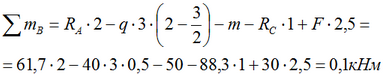Checking the supporting reactions of the beam

The verification of the found values ​​of the reactions of the supports can be carried out using the equation of the sum of the moments with respect to any other point, for example, B.If we substitute the obtained values ​​in the equation, the sum of the moments will be zero, then the support reactions have been found correctly.Note: In the equation of the sum of the moments, in contrast to the forces, the moments applied at the point relative to which the equation is made are always written down.
Video by subject (contains English subtitles)

Here, the value of 0.1kNm in the sum of the moments was obtained by rounding the values ​​of the found reactions to one decimal place, and shows the correctness of the calculations.

A positive sign of the magnitude of the support reactions suggests that their direction was originally chosen correctly.

If the found value of the reaction turns out to be negative, it must be directed in the opposite direction by changing the sign to «+».

Construction of Q and M diagrams for a beam >